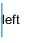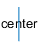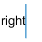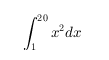# text

## 语法

text(x,y,txt)
text(x,y,z,txt)
text(___,Name,Value)
text(ax,___)
t = text(___)

## 说明

text(x,y,txt) 使用由 txt 指定的文本，向当前坐标区中的一个或多个数据点添加文本说明。若要将文本添加到一个点，请将 x 和 y 指定为标量。若要将文本添加到多个点，请将 x 和 y 指定为长度相同的向量。
text(x,y,z,txt) 在三维坐标中定位文本。

text(___,Name,Value) 使用一个或多个名称-值对组指定 Text 对象的属性。例如，'FontSize',14 将字体大小设置为 14 磅。您可以使用上述语法中的任意输入参数组合指定文本属性。如果您将 Position 和 String 属性指定为名称-值对组，则不需要指定 x、y、z 和 txt 输入。
text(ax,___) 将在由 ax 指定的笛卡尔坐标区、极坐标区或地理坐标区中创建文本，而不是在当前坐标区 (gca) 中创建文本。选项 ax 可以位于前面的语法中的任何输入参数组合之前。

t = text(___) 返回一个或多个文本对象。使用 t 修改所创建的文本对象的属性。有关属性和说明的列表，请参阅 Text 属性。可以使用上述任意语法指定一个输出。

## 示例

x = 0:pi/20:2*pi; y = sin(x); plot(x,y) text(pi,0,'\leftarrow sin(\pi)')x = linspace(-5,5); y = x.^3-12*x; plot(x,y) xt = [-2 2]; yt = [16 -16]; str = 'dy/dx = 0'; text(xt,yt,str)x = linspace(-5,5); y = x.^3-12*x; plot(x,y) xt = [-2 2]; yt = [16 -16]; str = {'local max','local min'}; text(xt,yt,str)plot(1:10) str = {'A simple plot','from 1 to 10'}; text(2,7,str)plot(1:10) str = {{'A simple plot','from 1 to 10'},'y = x'}; text([2 8],[7 7],str)plot(1:10) text(2,8,'A Simple Plot','Color','red','FontSize',14)x = linspace(-5,5); y = x.^3-12*x; plot(x,y) t = text([-2 2],[16 -16],'dy/dx = 0')t = 2x1 Text array: Text (dy/dx = 0) Text (dy/dx = 0) 

text 函数为每个文本说明创建一个文本对象。因此，t 包含两个文本对象。

t(1).Color = 'red'; t(1).FontSize = 14;## 输入参数

• 标量 - 将文本添加到单个点。

• 向量 - 将文本添加到多个点。

• 对于笛卡尔坐标区，第一个坐标是以数据单位表示的 x 轴上的位置。要更改单位，请设置 Text 对象的 Units 属性。

• 对于极坐标区，第一个坐标是以弧度表示的极坐标角 θ。

• 对于地理坐标区，第一个坐标是纬度（以度为单位）。

xy 的大小必须相同。

• 标量 - 将文本添加到单个点。

• 向量 - 将文本添加到多个点。

• 对于笛卡尔坐标区，第二个坐标是以数据单位表示的 y 轴上的位置。要更改单位，请设置 Text 对象的 Units 属性。

• 对于极坐标区，第二个坐标是以数据单位表示的半径。

• 对于地理坐标区，第二个坐标是经度（以度为单位）。

xy 的大小必须相同。

• 标量 - 将文本添加到单个点。

• 向量 - 将文本添加到多个点。

• 对于笛卡尔坐标区，第三个坐标是以数据单位表示的 z 轴上的位置。要更改单位，请设置 Text 对象的 Units 属性。

• 对于极坐标区和地理坐标区，第三个坐标会影响坐标区中文本的分层。

xyz 的大小必须相同。

#### 用于单个数据点的文本

• 使用元胞数组，其中每个元胞包含一行文本。例如，text(.5,.5,{'first','second'})

• 使用字符串数组，其中每个元素是一行文本。例如，text(.5,.5,["first","second"])

• 使用具有多个行的字符数组，其中每一行包含相同数量的字符。例如，text(.5,.5,['first '; 'second'])

• 使用 sprintf 创建一个换行符。例如，text(.5,.5,sprintf('first \nsecond'))

#### 希腊字母、特殊字符和数值变量

v = 42; txt = ['The value is ',num2str(v)];

### 名称-值对组参数

MATLAB 使用 FontWeight 属性从系统提供的字体中选择一种字体。并非所有字体都有加粗字体。因此，指定加粗字体仍可能得到普通字体。

• RGB 三元组是包含三个元素的行向量，其元素分别指定颜色中红、绿、蓝分量的强度。强度值必须位于 [0,1] 范围内，例如 [0.4 0.6 0.7]

• 十六进制颜色代码是字符向量或字符串标量，以井号 (#) 开头，后跟三个或六个十六进制数字，范围可以是 0F。这些值不区分大小写。因此，颜色代码 '#FF8800''#ff8800''#F80''#f80' 是等效的。

'red''r'[1 0 0]'#FF0000''green''g'[0 1 0]'#00FF00''blue''b'[0 0 1]'#0000FF''cyan' 'c'[0 1 1]'#00FFFF''magenta''m'[1 0 1]'#FF00FF''yellow''y'[1 1 0]'#FFFF00''black''k'[0 0 0]'#000000''white''w'[1 1 1]'#FFFFFF''none'不适用不适用不适用无颜色

RGB 三元组十六进制颜色代码外观
[0 0.4470 0.7410]'#0072BD'[0.8500 0.3250 0.0980]'#D95319'[0.9290 0.6940 0.1250]'#EDB120'[0.4940 0.1840 0.5560]'#7E2F8E'[0.4660 0.6740 0.1880]'#77AC30'[0.3010 0.7450 0.9330]'#4DBEEE'[0.6350 0.0780 0.1840]'#A2142F''left'（默认值）'center''right''data'（默认值）数据坐标。
'normalized' 针对坐标区进行归一化。坐标区的左下角映射到 (0,0)，右上角映射到 (1,1)
'inches'英寸。
'centimeters'厘米。
'characters'

• 字符宽度 = 字母 x 的宽度。

• 字符高度 = 两个文本行的基线之间的距离。

'points'磅。一磅等于 1/72 英寸。
'pixels'

• 在 Windows 系统上，一个像素是 1/96 英寸。

• Macintosh 系统上，一个像素是 1/72 英寸。

• 'tex' - 使用 TeX 标记子集解释字符。

• 'latex' - 使用 LaTeX 标记解释字符。

• 'none' - 显示字面字符。

#### TeX 标记

^{ }上标'text^{superscript}'
_{ }下标'text_{subscript}'
\bf粗体'\bf text'
\it斜体'\it text'
\sl伪斜体（通常与斜体相同）'\sl text'
\rm常规字体'\rm text'
\fontname{specifier}字体名称 - 将 specifier 替换为字体系列的名称。您可以将此说明符与其他修饰符结合使用。'\fontname{Courier} text'
\fontsize{specifier}字体大小 - 将 specifier 替换为以磅为单位的数值标量值。'\fontsize{15} text'
\color{specifier}字体颜色 - 将 specifier 替换为以下颜色之一：redgreenyellowmagentablueblackwhitegraydarkGreenorangelightBlue'\color{magenta} text'
\color[rgb]{specifier}自定义字体颜色 - 将 specifier 替换为三元素 RGB 三元组。'\color[rgb]{0,0.5,0.5} text'

\alpha

α

\upsilon

υ

\sim

~

\angle

\phi\leq

\ast

*

\chi

χ

\infty

\beta

β

\psi

ψ

\clubsuit

\gamma

γ

\omega

ω

\diamondsuit

\delta

δ

\Gamma

Γ

\heartsuit

\epsilon

ϵ

\Delta

Δ

\spadesuit

\zeta

ζ

\Theta

Θ

\leftrightarrow

\eta

η

\Lambda

Λ

\leftarrow

\theta

θ

\Xi

Ξ

\Leftarrow

\vartheta

ϑ

\Pi

Π

\uparrow

\iota

ι

\Sigma

Σ

\rightarrow

\kappa

κ

\Upsilon

ϒ

\Rightarrow

\lambda

λ

\Phi

Φ

\downarrow

\mu

µ

\Psi

Ψ

\circ

º

\nu

ν

\Omega

Ω

\pm

±

\xi

ξ

\forall

\geq

\pi

π

\exists

\propto

\rho

ρ

\ni

\partial

\sigma

σ

\cong

\bullet

\varsigma

ς

\approx

\div

÷

\tau

τ

\Re

\neq

\equiv

\oplus

\aleph

\Im

\cup

\wp

\otimes

\subseteq

\oslash

\cap

\in

\supseteq

\supset

\lceil

\subset

\int

\cdot

·

\o

ο

\rfloor

\neg

¬

\nabla

\lfloor

\times

x

\ldots

...

\perp

\surd

\prime

´

\wedge

\varpi

ϖ

\0

\rceil

\rangle

\mid

|

\vee

\langle

\copyright

#### LaTeX 标记## 输出参数

Text 对象。在创建特定 Text 对象后，使用 t 的元素对其进行修改。有关属性列表，请参阅 Text 属性

## 提示

• 默认情况下，文本对象的 Clipping 属性设置为 'off'，这样文本可能显示在坐标区之外。若要裁剪文本使其在坐标区边界内，请将该属性设置为 'on'